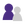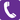## Answered By: Lana Alsabbagh Last Updated: Jun 21, 2015     Views: 31

Uses an algorithm where "h" represents a constant value and then counts determines the median number of cites within that "h" number of cites. he “h” is a constant number used through out the measure derived from the entire publication period of a publication. The h5 number is the same constant number, except only articles of the last five years are used.

The h-index indicates that at least “h” number of articles from the journal were cited “h” number of times and h is the highest possible number. To use the example from the Metrics page of Google Scholar (http://scholar.google.com/intl/en/scholar/metrics.html#metrics), the reason the h-index number was 3 is that there are at least three articles were cited at least three times. The index could not be 4 because there are not 4 articles that had at least 4 cites.

The h-core is based on the h-index number. It is designed to show that even though the h-index number was “3”, the top 3 (or whatever the h-index value was) are used for determining the metrics that follow.

The h-median is the medium number of citations from the citations that make up the h-core. It is not the average of the citations (which would be 10.6 ((17+9+6) /3))). It is the number of the publication that sits in the exact middle of the h-core list (no word on what they do if the h-index number is even).

Therefore, to interpret the data for law journals from Google Scholar.

Columbia Law Journal has had at least 41 articles that were cited at least 41 times in the past five years. The median number of cites to those 41 articles was 55 (meaning that 20 articles had more than 55 cites, 20 had less and one had 55.

Harvard Law had at least 40 articles cited 40 times in the past five years. The median number was 66

Roughly comparing the two, Columbia had one more articles cited, but the articles in Harvard were cited more often.

Remember, you can’t trust statistics to give you the full picture.In person Visit the Reference Help Desk at the LibraryPhone us: 4403-4055 / 6338Click here to find answers of Frequently Asked Questions